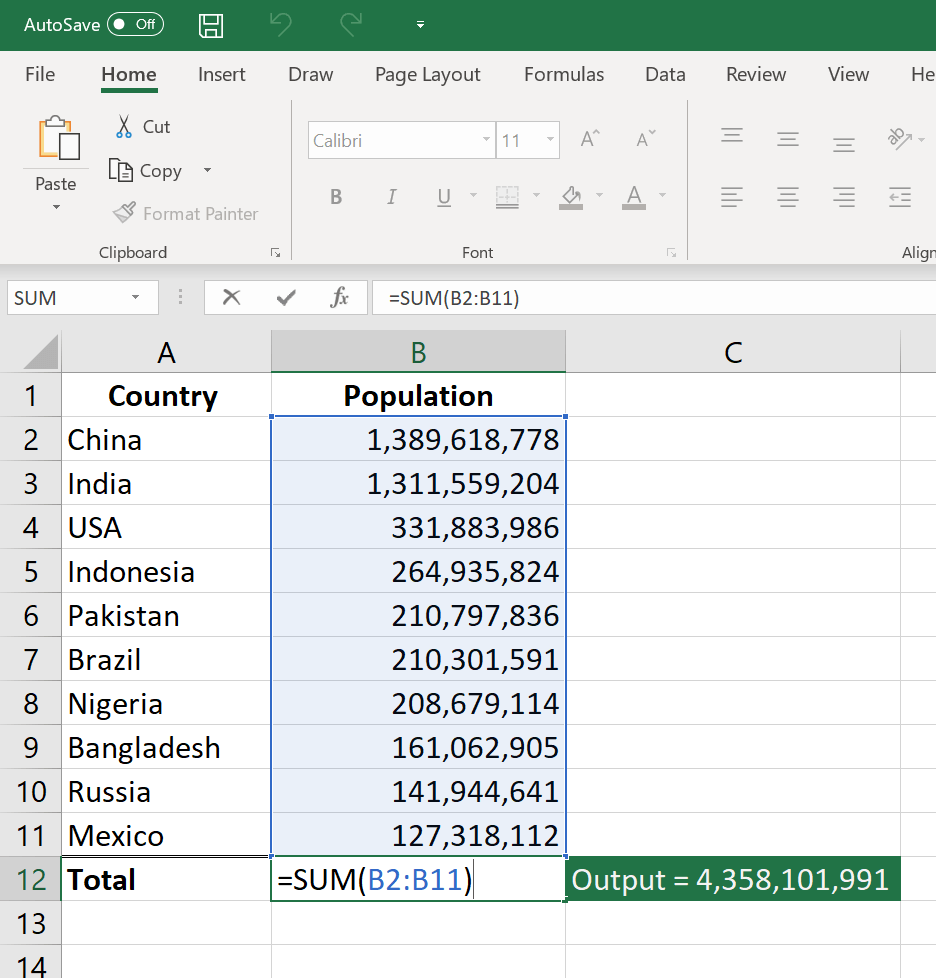# Basic Operations in MS Excel.

Microsoft Excel is a software program produced by Microsoft that allows users to organize, format and calculate data with formulas using a spreadsheet system. This software is part of the Microsoft Office suite and is compatible with other applications in the Office suite.

### Arithmetic Precedence

Microsoft Excel follows the rules of arithmetic precedence when evaluating formulas.

 ( ) operations enclosed in parentheses are evaluated first; nested parentheses are evaluated from the inside out ^ exponentiation * and / multiplication and division, evaluated from left to right + and – addition and subtraction, evaluated from left to right

Examples 1: the formula =5*3-4^2 evaluates as -1. Example 2: the formula =(5*(3-4)^2) evaluates as 5

### Basic Excel Formulas For Your Workflow

Excel formula is always entered by referencing the cell that contains the data you want to calculate. The first cell is known as cell A1, because is under column A and in row 1.

1. SUM: The SUM function is the first must-know formula in Excel. It usually aggregates values from a selection of columns or rows from your selected range.

=SUM(number1, [number2], …)

Example:

=SUM(B2:G2) – A simple selection that sums the values of a row.

=SUM(B2:B8) – A simple selection that sums the values of a column.

=SUM(A2:A7, A9, A12:A15) – A sophisticated collection that sums values from range A2 to A7, skips A8, adds A9, jumps A10 and A11, then finally adds from A12 to A15.

=SUM(A2:A8)/20 – Shows you can also turn your function into a formula.Another SUM example is =(B2+B3+B4+B5+B6+B7+B8)

Subtraction example is =(B2-B3)

Multiplication example is =(B4*B5)

Division example is =(B7/2)

2. AVERAGE: The AVERAGE function should remind you of simple averages of data such as the average number of shareholders in a given shareholding pool.

=AVERAGE(number1, [number2], …)

Example: =AVERAGE(B2:B11) – Shows a simple average, also similar to (SUM(B2: B11)/10)

3. COUNT: The COUNT function counts all cells in a given range that contain only numeric values.

=COUNT(value1, [value2], …)

Example: COUNT(A:A) – Counts all values that are numerical in A column. However, you must adjust the range inside the formula to count rows.

COUNT(A1:C1) – Now it can count rows.

4. IF: The IF function is often used when you want to sort your data according to a given logic. The best part of the IF formula is that you can embed formulas and function in it.

=IF(logical_test, [value_if_true], [value_if_false])

Example: =IF(C2<D3, ‘TRUE,’ ‘FALSE’) – Checks if the value at C3 is less than the value at D3. If the logic is true, let the cell value be TRUE, else, FALSE

=IF(SUM(C1:C10) > SUM(D1:D10), SUM(C1:C10), SUM(D1:D10)) – An example of a complex IF logic. First, it sums C1 to C10 and D1 to D10, then it compares the sum. If the sum of C1 to C10 is greater than the sum of D1 to D10, then it makes the value of a cell equal to the sum of C1 to C10. Otherwise, it makes it the SUM of C1 to C10.

5. MAX & MIN: The MAX and MIN functions help in finding the maximum number and the minimum number in a range of values.

=MIN(number1, [number2], …)

Example: =MIN(B2:C11) – Finds the minimum number between column B from B2 and column C from C2 to row 11 in both column B and C.

=MAX(number1, [number2], …)

Example: =MAX(B2:C11) – Similarly, it finds the maximum number between column B from B2 and column C from C2 to row 11 in both column B and C.

Lesson tags: Tech003
Back to: Computer Application Packages for Beginner.